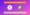# UGC NET Data Interpretation Notes Notes 2020 | Paper 1 Data Interpretation NotesUGC NET Data Interpretation Notes 2020: Are your preparing for UGC NET Paper 1? Do you have the latest Data Interpretation Notes?

Don’t worry! Here we have provided the latest UGC NET Data Interpretation Notes.

You can check easily check the Data Interpretation Notes and also able to download the PDF.

## UGC NET Data Interpretation Notes 2020

UGC NET Exam, the exam which tests the eligibility of Indian Nationals for ‘Assistant Professor’ or for ‘Junior Research Fellowship and Assistant Professor’ both in Indian Universities and Colleges.

Before starting a complete guide on UGC NET Paper 1 Syllabus 2020 for Environmental Science, you should have an overview of the exam.

UGC NET 2020 June Exam Overview

Here you can check the UGC NET exam overview:

 Exam Name University Grants Commission National Eligibility Test (UGC NET) Conducting Body National Test Agency (NTA) Exam Mode Computer Based Test (CBT) Exam Duration 3 hours Application Mode Online Official Website ugcnet.ntanet.nic.in

Here you can check the complete notes on Data Interpretation.

UGC Data Interpretation Notes cover the topics as given below.

• Sources, Acquisition and Interpretation of Data.
• Quantitative and Qualitative Data.
• Graphical Representation and Mapping of Data.

### Types Data set in UGC NET Exam

You should know that the Data can be written or represented in 4 Forms. These are,

• Numerical:- Data in the numerical form
• Table Form:- Data in Tabular Form
• Mixed form:- Data in Mix Form
• Graphical form Like Line, Bar graph, PI Chart etc.

### Important Information Related To UGC Data Interpretation Questions

• The questions will not only come from quantitative skills but also relative, comparative and analytical ability.
• Mainly you have to find a relationship between the two tables or graphs before attempting the questions.
• You will find the Data Interpretation questions are based on the information given in tables and graphs. These questions test your ability to interpret the information presented and to select the appropriate data for answering a question
• Get a general picture of the information before reading the question.
• Avoid lengthy calculations generally, data interpretation questions do not require to do extensive calculations and computations. Most questions simply require reading the data correctly and carefully.
• Breakdown lengthy questions into smaller parts and eliminate impossible choices.
• Answer the questions asked and not what you think the questions should be.
• Tables are often used in reports, magazines, and newspapers to present a set of numerical facts.

### Other Important Sections

Percentage and Ratio applications: These two chapters from Quantitative Aptitude has a certain role to be played in DI calculation.

• Master your Percentage, Ratio, Profit and Loss, Average, Interest Calculations
• Some other necessary things are learned multiplication tricks for three/four digits, Square, Cube.
• Avoid Unnecessary Calculation

Approximation techniques: A very large number of these questions check your ability to compare or calculate fractions and percentages. If you sit down to actually calculate the answer, you would end up spending more time than required. Here are a few ideas that you can use for approximation.

• Learn the concept of Approximations and Estimations. These are very important to solve DI questions very fast.
• When trying to calculate (approximate) a fraction p/q, add value to the denominator and corresponding value to the numerator before calculating (approximating).
• If the percentage growth rate is r for a period of t years, the overall growth rate is approximate: rt + t * (t-1) * r2 / 2. Follow RS Aggrawal Book

Calculation Techniques specific to the data sets: There are calculation techniques especially in case of Pie Chart, Line Chart and Bar chart that one should know to reduce the calculation time.

Pie Chart is a circular form of Data representation. In this, the circle is divided into sectors either percentwise or degree-wise.

In the per cent-wise division, the total area of the chart is taken to be 100% and in degrees division, the total area of the chart is taken to be 360 degrees.

### Other Important UGC NET Paper 1 Notes

You should have the following study materials to boost your exam preparation for the NTA UGC NET exam.

Click on the link to access other important notes related to the UGC NET Paper 1 exam.

We have covered the detailed guide on UGC NET Data Interpretation Notes 2020. Feel free to ask us any questions in the comment section below.